The BTL Procedure

REPEATED <repeated-effect> < / options> ;

The REPEATED statement is used to specify the
matrix in the mixed model. Its syntax is different from that of the REPEATED statement in PROC GLM. If no REPEATED statement is specified,is assumed to be equal to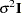.

For many repeated measures models, no repeated effect is required in the REPEATED statement. Simply use the SUBJECT= option to define the blocks ofand use the TYPE= option to define their covariance structure. In this case, the repeated measures data must be similarly ordered for each subject, and you must indicate all missing response variables with periods in the input data set unless they all fall at the end of a subject’s repeated response profile. These requirements are necessary in order to inform PROC BTL of the proper location of the observed repeated responses.

Specifying a repeated effect is useful when you do not want to use periods to indicate missing values in the input data set. The repeated effect must contain only classification variables. Make sure that the levels of the repeated effect are different for each observation within a subject; otherwise, PROC BTL constructs identical rows incorresponding to the observations with the same level. This results in a singularand an infinite likelihood.

Whether you specify a REPEATED effect or not, the rows offor each subject are constructed in the order in which they appear in the input data set.

You can specify the following options in the REPEATED statement after a slash (/).

GROUP=effect
GRP=effect

defines an effect specifying heterogeneity in the covariance structure of. All observations having the same level of the GROUP effect have the same covariance parameters. Each new level of the GROUP effect produces a new set of covariance parameters with the same structure as the original group. You should exercise caution in properly defining the GROUP effect, because strange covariance patterns can result from its misuse. Also, the GROUP effect can greatly increase the number of estimated covariance parameters, which can adversely affect the optimization process.

Continuous variables are permitted as arguments to the GROUP= option. PROC BTL does not sort by the values of the continuous variable; rather, it considers the data to be from a new subject or group whenever the value of the continuous variable changes from the previous observation. Using a continuous variable decreases execution time for models with a large number of subjects or groups and also prevents the production of a large "Class Levels Information" table.

LDATA=SAS-data-set

reads the coefficient matrices associated with the TYPE=LIN(number ) option. The data set must contain the variables PARM, ROW, COL1–COLn, or PARM, ROW, COL, VALUE. The PARM variable denotes which of the number coefficient matrices is currently being constructed, and the ROW, COL1–COLn, or ROW, COL, VALUE variables specify the matrix values, as they do with the RANDOM statement option GDATA=. Unspecified values of these matrices are set equal to 0.

LOCAL
LOCAL=EXP(<effects> )
LOCAL=POM(POM-data-set )

requests that a diagonal matrix be added to. With just the LOCAL option, this diagonal matrix equals, and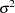becomes an additional variance parameter that PROC BTL profiles out of the likelihood provided that you do not specify the NOPROFILE option in the PROC BTL statement. The LOCAL option is useful if you want to add an observational error to a time series structure (Jones and Boadi-Boateng 1991) or a nugget effect to a spatial structure (Cressie 1991).

The LOCAL=EXP(<effects> ) option produces exponential local effects, also known as dispersion effects, in a log-linear variance model. These local effects have the form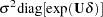where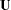is the full-rank design matrix corresponding to the effects that you specify, and
are the parameters that PROC BTL estimates. An intercept is not included inbecause it is accounted for by. PROC BTL constructs the full-rankin terms of 1s and1s for classification effects. Be sure to scale continuous effects insensibly.

The LOCAL=POM(POM-data-set ) option specifies the power-of-the-mean structure. This structure possesses a variance of the form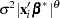for theth observation, where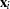is theth row of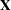(the design matrix of the fixed effects), and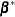is an estimate of the fixed-effects parameters that you specify in POM-data-set.

The SAS data set specified by POM-data-set contains the numeric variable Estimate (in previous releases, the variable name was required to be EST), and it has at least as many observations as there are fixed-effects parameters. The first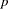observations of the Estimate variable in POM-data-set are taken to be the elements of, whereis the number of columns of. You must order these observations according to the non-full-rank parameterization of the BTL procedure.

LOCALW

specifies that only the local effects and no others be weighted. By default, all effects are weighted. The LOCALW option is used in connection with the WEIGHT statement and the LOCAL option in the REPEATED statement.

NONLOCALW

specifies that only the nonlocal effects and no others be weighted. By default, all effects are weighted. The NONLOCALW option is used in connection with the WEIGHT statement and the LOCAL option in the REPEATED statement.

SUBJECT=effect
SUB=effect

identifies the subjects in your mixed model. Complete independence is assumed across subjects; therefore, the SUBJECT= option produces a block-diagonal structure inwith identical blocks. When the SUBJECT= effect consists entirely of classification variables, the blocks ofcorrespond to observations sharing the same level of that effect. These blocks are sorted according to this effect as well.

Continuous variables are permitted as arguments to the SUBJECT= option. PROC BTL does not sort by the values of the continuous variable; rather, it considers the data to be from a new subject or group whenever the value of the continuous variable changes from the previous observation. Using a continuous variable decreases execution time for models with a large number of subjects or groups.

If you want to model nonzero covariance among all of the observations in your SAS data set, specify SUBJECT=INTERCEPT to treat the data as if they are all from one subject. However, be aware that, in this case, PROC BTL manipulates anmatrix with dimensions equal to the number of observations. If no SUBJECT= effect is specified, then every observation is assumed to be from a different subject andis assumed to be diagonal. For this reason, you usually want to use the SUBJECT= option in the REPEATED statement.

TYPE=covariance-structure

specifies the covariance structure of thematrix. The SUBJECT= option defines the blocks of, and the TYPE= option specifies the structure of these blocks. The default structure is VC. See PROC MIXED for details about the available covariance structures.

Note: This procedure is experimental.Previous Page | Next Page | Top of Page